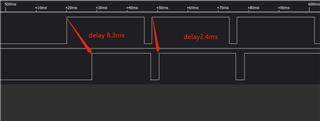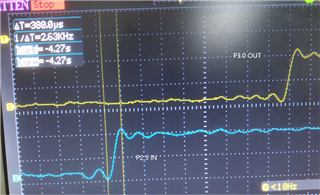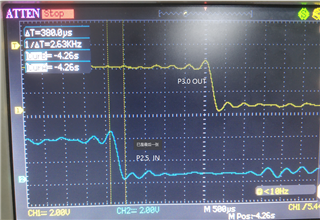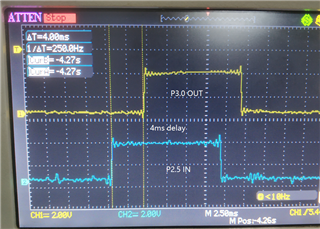If you have a related question, please click the "Ask a related question" button in the top right corner. The newly created question will be automatically linked to this question.

# MSP430F2132: IO signal changes, slow response of internal registers and interrupts

Part Number: MSP430F2132

Hello！

I need to output IO signal or PWM immediately when the input IO signal changes. However, it is found that the response delay is severe, about 1.4-6ms。

simple.The code is as follows:

```void main(void)
{

WDTCTL = WDTPW + WDTHOLD; // Stop WDT

P2DIR|=BIT5;
// P2REN&=~BIT5;
P2IES&=~BIT5;
P2OUT&=~BIT5;
P2IFG&=~BIT5;
P2DIR&=~BIT5;
//P2IE|=BIT5;

//p3.0 trigger out
P3DIR |= BIT0;
//P3REN|=BIT0;

while(1){
if(P2IN&BIT5) //p2.5: mcu-triggerIn
{
P3OUT|=BIT0;
}else{
P3OUT&=~BIT0;
}
}

}
```

or

```void main(void)
{

WDTCTL = WDTPW + WDTHOLD; // Stop WDT

//P2.5 interrupt
P2DIR|=BIT5;
// P2REN&=~BIT5;
P2IES&=~BIT5;
P2OUT&=~BIT5;
P2IFG&=~BIT5;
P2DIR&=~BIT5;
//P2IE|=BIT5;

//p3.0 trigger out
P3DIR |= BIT0;
//P3REN|=BIT0;

_EINT();
__low_power_mode_1();
}

//mcu-triggerin
#pragma vector=PORT2_VECTOR
__interrupt void Port_2(void)
{

P2IFG&=~(BIT5 | BIT4);
if(P2IN&BIT5) //p2.5: mcu-triggerIn
{
P3OUT|=BIT0;
P2IES|=BIT5;

}else{
P3OUT&=~BIT0;
P2IES&=~BIT5;
}

}```

The logic levels of input and output ports are monitored externally, and the delay is found, and the delay time is related to the duty cycle of input level and long waiting time.I think the possible reasons are:
1) The external input level changes and it is slow to update the internal pin register
2) The change from output IO to drive IO level is slow

• Hello Jun Zhang,

To get a faster response time, increasing the CPU speed will reduce the latency of the response. Also, it would be recommended to use the interrupt version versus the polling version. This will allow the program to react faster with the ISR instead of having to react when it reaches that specific line of code in the polling version.

sincerely,

Luke

• I don't doubt the speed of MCU code execution. The dominant frequencies of 1m and 16m are the same. The delay time between input level and output level is large（1.5ms ~ 8ms）
I suspect one or two of the following:
1) After the input level changes, the change delay of the driving pin register
2) After the pout register value changes, the drive output level is delayed

• The delay time between input level and output level is large（1.5ms ~ 8ms）

Your (assumed LSA) capture of the input and output signals doesn't show the rise and fall times of the input signal.

Have you got an oscilloscope as that would allow the rise and fall times to be seen? Possibly a slow rise or fall time could explain the apparent delay.

Also, what device is the source of the input signal?

Looking at the code I can't see anything which explains the delay.

• thanks

the source of the input signal：Other MSP430 periodically output signals

P2.5 input change, synchronous output P3.0 through IO interrupt

P2.5 rise time ≈  P3.0  rise time =380us

P2.5 fall time ≈  P3.0  fall time =370us

p2.5 rise - p3.0 rise  delay：4ms

p2.5 fall - p3.0 fall  delay：2.6ms

```
void main(void)
{

WDTCTL = WDTPW + WDTHOLD; // Stop WDT

P2DIR|=BIT5;
//P2REN&=~BIT5;
P2OUT&=~BIT5;
P2IFG&=~BIT5;
P2DIR&=~BIT5;
P2IES&=~BIT5;
P2IE|=BIT5;

//p3.0 trigger out
P3DIR |= BIT0;
P3OUT&=~BIT0;

_EINT();
//__low_power_mode_1();
}

//mcu-triggerin
#pragma vector=PORT2_VECTOR
__interrupt void Port_2(void)
{

P2IFG&=~BIT5;
if(P2IN&BIT5) //p2.5: mcu-triggerIn
{
P3OUT|=BIT0;
P2IES|=BIT5;

}else{
P3OUT&=~BIT0;
P2IES&=~BIT5;
}

}```• After changing the input IO port, it is found that the response is fast. After analysis, it turns out that pin2.5 has a large capacitance filter. After adjustment, the reaction time is fast.